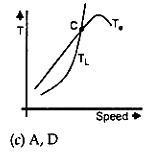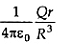# The dc motor, which can provide zero speed regulation at full load without any controller, is

1.  series

2.  shunt

3.  cumulative compound

4.  differential compound

4

shunt

Explanation :
No Explanation available for this question

# The probes of a non - isolated, two - channel oscilloscope are clipped to points A, B and C in the circuit of the adjacent figure. Vin is a square wave of a suitable low frequency. The display on Ch1 and Ch2 are as shown on the right. Then the "Signal" and "Ground" probes S1, G1 and S2, G2 of Ch1 and Ch2 respectively are connected to points.

1.  A, B, C, A

2.  A, B, C, B

3.  C, B, A, B

4.  B, A, B, C

4

A, B, C, B

Explanation :
No Explanation available for this question

# A single phase full-wave half-controlled bridge converter feeds an inductive load. The two SCRs in the converter are connected to a common DC bus. The converter has to have a freewheeling diode

2.  because the converter does not provide for free-wheeling for high values of triggering angles

3.  or else the free-wheeling action of the converter will cause shorting of the AC supply

4.  or else if a gate pulse to one of the SCRs is missed, it will subsequently cause a high load current in the other SCR

4

because the converter does not provide for free-wheeling for high values of triggering angles

Explanation :
No Explanation available for this question

# The electromagnetic torque Te of a drive, and its connected load torque TL are as shown below. Out of the operating points A, B, C and D, the stable ones are

1.

2.

3.

4.

4Explanation :
No Explanation available for this question

# "Six MOSFETs connected in a bridge configuration (having no other power device) MUST be operated as a Voltage Source Inverter (VSI)". This statement is

1.  True, because being majority carier devices, MOSFETs are voltage driven

2.  True, because MOSFETs have inherently anti-parallel diodes

3.  False, because it can be operated both as Current Source Inverter (CSI) or a VSI

4.  False, because MOSFETs can be operated as excellent constant current sources in the saturation region

4

False, because MOSFETs can be operated as excellent constant current sources in the saturation region

Explanation :
No Explanation available for this question

# The input signal Vin shown in the figure is a 1 kHz square wave voltage that alternates between +7V and -7V with a 50% duty cycle. Both transistors have the same current gain, which is large. The circuit delivers power to the load resistor RL. What is the efficiency of this circuit for the given input Choose the closest answer.

1.  46 %

2.  55 %

3.  63 %

4.  92 %

4

92 \%

Explanation :
No Explanation available for this question

# A, B, C and D are input bits, and Y is the output bit in the XOR gate circuit of the figure below. Which of the following statements about the sum S of A, B, C, D and Y is correct

1.  S is always either zero or odd

2.  S is always either zero or even

3.  S = 1 only if the sum of A, B, C and D is even

4.  S = 1 only if the sum of A, B C and D is odd

4

S = 1 only if the sum of A, B C and D is odd

Explanation :
No Explanation available for this question

# The differential equation dx/dt = ((1 - x)/τ) is discretised using Euler's numerical integration method with a time step T > 0. What is the maximum permissible value of ?T to ensure stability of the solution of the corresponding discrete time equation?

1.  1

2.  τ/2

3.  τ

4.  2τ

4

Explanation :
No Explanation available for this question

# The switch S is the circuit of the figure is initially closed. It is opened at time t = 0. You may neglect the Zener diode forward voltage drops. What is the behaviour of VOUT for t > 0

1.  It makes a transition from - 5 V to + 5 V at t = 12.98 µs

2.  It makes a transition from - 5 V to + 5 V at t = 2.57 µs

3.  It makes a transition from + 5 V to - 5 V at t = 12.98 µs

4.  It makes a transition from + 5 V to - 5 V at t = 2.57 µs

4

It makes a transition from + 5 V to - 5 V at t = 2.57 µs

Explanation :
No Explanation available for this question

# A solid sphere made of insulating material has a radius R and has a total charge Q distributed uniformly in its volume. What is the magnitude of the electric field intensity, E, at a distance r (0 < r < R) inside the sphere

1.

2.

3.

4.

4